The Gravitation Conception and Experiments

Alexander V. Frolov
P.O.Box 37, St.-Petersburg, 193024, Russia

Introduction

By Newton's laws and by Nature common sense any  active force can be created only in pair with reactive force. This rule describe the balanced interaction between two bodies. But there is a very interesting case: one body and some substance around this body, air or the ether.

In  empty space (vacuum) the body can be considered as localized in space energy that is not localized in time and the substance energy (ether) can be considered as non-localized  (dispersed) in space but it is localized in time. This symmetry for spatial or chronal localization  is described by Heizenberg's equations. In  this case the conservation law of energy for the "one-body closed system" can be represented as balance of mass-form energy process (the body) and corresponding to this mass the space-time energy process (the ether). It is local space-time of the body. By this concept, there is no any physical sense in notion of space-time if it is not connected with some certain material object. Any mass object is some process and there is its local space-time process.

According to the Newton's 1670th notion for the ether, there is no any ether in open space (space quite far from any mass) but the ether exist only inside and near the bodies . By this concept for the ether it will possible to develop the ether-dynamics and it will be demonstrated in this paper. The key to understanding of the nature of the gravitation, by Academician Vavilov, who is the author of the book about Sir Isaac Newton [1, p.84], is the gradient of the density of the ether in a matter and empty space.

Just conservation law is the reason for supposition about chronal effect. The effect should be
demonstrated when the system use a transformation of different forms of energy (mass-form and time-form) to produce the non-reactive propulsion force or free power generation. One of such sort system is so called inertioid.  Let us take this example to disclose the nature of inertia.

By proposed above formulation, known Newton's law describes the balance of different energy forms that is demonstrated itself as reaction for any action. It is known that in empty space (in vacuum) any  accelerating or decelerating body have some reactive interaction with space: it is inertia effect. So, space itself can be represented as some substance. Also there is the equality of the weight mass and inertial mass that confirm this supposition.

In the paper "Optic" [1, p.86] Sir Isaac Newton stated that there is no the ether in "open space". If we are considering the mass-particle  as "condensed ether", around the mass the ether is more rarefied, since it was used to create the particles, but outside the body the density of the ether is increasing with distance from the body. In this case,  the "open space" must be considered as a very dense ether, but by Newton  there  is no ether in the "open space". How we can connect all this together?

Perhaps, solution is the understanding of the structure of the space, i.e. the ether can be
considered as some kind change of the structure of the vacuum. Some local change of vacuum structure that produce the mass-particle must be compensated by corresponding opposite change of vacuum structure around the mass-particle. The gradient of the density of the ether is the reason for ether flows, by Newton, and it can be considered in other words as gradient of potential and some potential field. In this case, the notion of the "density of the ether" can be changed to the notion of the gradient of the potential: "gradient ".

Taking that "ether" and " gradient " is the same notion, we avoid showed  above question, since
the gradient is increasing if the distance is increasing also, but for any two point of the open space (quite far from any mass) there is no any gradient of potential. So, the ether can not be considered as some material substance separately from space that is produced by some mass. Note that any matter also can not be considered separately from space. Matter is only special structure of space or space is only different forms of the matter and it is connected with some energy value per unit of space volume.  Important part of the gravitation concept  that is proposed above, is follows from the  M. Faraday's opinion about  matter  nature. In his letter of June 25, 1844 to Richard Tailor, M. Faraday wrote: So, matter anywhere is continuous... it is not necessary to suppose the difference between atoms of the matter and some intermediate space. The forces around centers provide to these centers the properties of the matter...

M.Faraday considered this aspect to make conclusion for nature of electric conductivity and
insulation. But his point of view can be developed for gravity aspect also.  Atom and space around of atom is the same matter in different states. Atom or element of the atom is local change of space structure only.

Pre-conditions above are basis for conclusion: Any body displace ether mass that equal to the body mass, it is well known Arhimed's law for ether ( in generalized form). Since  motion of any body have place inside of real substance,  this substance (space-time itself) can be used for "reactionless" or etherdynamics (like aerodynamical) method of motion. Forces are acting to the body in this case are external and law of conservation (for energy, momentum, impulse) is true if body and substance around body (air, liquid, ether)  is considered as closed system.

Since ether-dynamical motion use mass of ether (that was demonstrated as inertial mass) to
create the ether gradient, in this case inertia effect is not producing for accelerating or decelerating
object, so:  the ether-dynamical reactionless motion is inertia-less motion.

Important aspect of the gravitation concept described in this paper is notion of paired processes of nature: gravitation - radiation. Joseph Hasslberger described this point of view in details ( "A New Beginning for Thermodynamics", of May 8, 1993). Two paired processes are created in Nature in strength of the energy balance, conservation law. So, if the radiation is emission of the photons (electromagnetic waves of any wave-length) from mass object, the gravitation is absorption of the photons by mass objects. Any material object is radiating body (heat flow) and it is "gravitating" body (cold  flow).

In other words, phenomenon of material mass as source of gravitation field can be presented  as absorption and transformation of electromagnetic waves. The source of these waves are known as zero-point fluctuations. Concept on the zero-point electromagnetic energy fluctuations those exist in empty space is published by A. Sakharov, H. Puthoff, A. Rueda, B. Haisch ,,,.

On the base of the above let's make  some proposals for experiments and consider the results
of some real experiments.

Motion Inside of the Substance

Well-known case of the motion inside of the substance is propulsion force described as
Magnus effect ( Henrick G.  Magnus), discovered in 1852, Figure 1.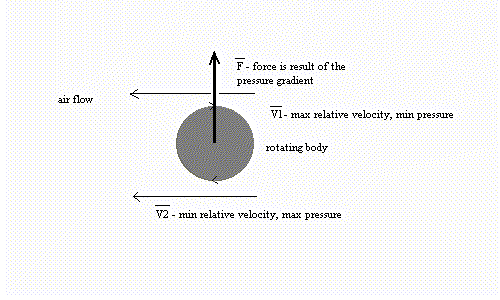Fig.1

Other well-known method for the same aerodynamical effect is Gukovsky-Chaplygin wing
profile, Fig.2.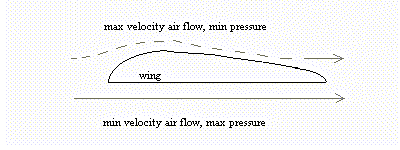Fig.2

Different relative velocities between body (cylinder or wing)  and space substance (air, liquid...) correspond to different pressure values.  There is some non-compensated  resulting force F that is described as gradient of pressure.  This force is not reactive and  it can be considered as gravitation force.

Now let's consider magnetic field B1 ( constant field of some source ) and magnetic field of
some moving charged mass Mq  ( magnetic field of the current ) B2, Fig.3.Fig.3

From one side of the charged moving mass q the  fields are co-directed  but from other side they are contra-directed.  Result field around charged mass q is asymmetrical. In strength of this reason well-known Lorenz force F is result of the gradient magnetic field i.e. it is the different ether pressure on the moving charged mass-body.  This aspect was not disclosed before since modern science don't recognized the ether concept. It is necessary to provide the space with properties of substance for it. In this sense Ether concept is true and ether gradient can be considered as gradient of space energy density or density of space itself.

So, nature of Lentz force and Magnus aerodynamical effect the same: self-propulsion non-
reactive force is result of the gradient of energy in space round the body. Since the energy by definition is description of the different types of the motion, the gradient above (for electromagnetic version also) is result of relative velocity only. Since it lead to gravitation type propulsion,  the conclusion can be formulated: phenomenon of gravitation and time can be described as phenomenon of relative motion.

Note that this force can be created in closed system also.  Figure 2 show that structure of space ( or structure of liquid, gas, ether and so on) is changed only in area near of the wing and it create the pressure gradient. So, in big volume of space for any closed system this situation can be created also. It is clear from the thought experiment, for example, there is an airplane in some big box. If the airplane is in the rest state, total weight of the box will include the weight of the airplane. But if the airplane is in flight (along some circumference trajectory inside of the box, for example),  weight of the box don't include the weight of airplane. When the airplane made the stop, the weight of the box must be increased. So, if the box is "black box" and we can not to observe the situation inside of it, the changes of the box weight lead to conservation law violation from the point of view that use the local frame of reference (box). But in the global frame of references the flying airplane is in interaction with the ether but not only with air. The air is material particles are made from the ether and they are connected with the ether like any atom is connected with its surrounding space-time. The interaction between the airplane and the ether is real antigravity technology. To change connection "airoplan-air-ether" into "spacecraft-ether" it is necessary to create the gradient of the ether pressure instead of the air pressure gradient.

The Asymmetry of Electric Energy

Space has own energy balance (mass-form and time-form) in certain volume of space but we
can change it by means of electric energy, for example.  Simple example is dielectric body motion in potential electric field of some charged mass, Fig.4. This experiment can be demonstrated by any charged thing ( pen or comb ) that attract a small paper pieces. The potential field E created by charged mass is result of potential gradient because potential is decreasing if distance from charged body is increasing; intensity E = - grad phi.
Note: It is not special gradient field between two charged bodies; this gradient is provided by property of space only.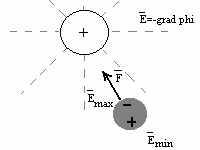Fig. 4

Own field of charged mass is symmetrical relative of its center. In strength of this reason all forces to mass M are balanced and there is no propulsion force. But when own field of charged mass is asymmetrical relatively mass itself (in strength of the special surface), the body can create some gradiental field and non-compensated force for itself.

Other method to create reactionless propulsion force by means of the field asymmetry is known as Biffeld-Brown effect. By T.T.Brown's patent number 300,311 of 15 Nov.1928, priority of 15 Aug.1927, the nature of the force "X" is not disclosed by author. This force is created in dielectric of capacitor and it is directed to positive charged plate. Some explanation for this force is proposed here.

Let's consider the method for non-reactive propulsion that use centrifugal force acceleration. In the case of the circumference trajectory, force F=ma is symmetrical (the value of force is constant in any point of the trajectory since the acceleration is constant). If the trajectory is asymmetrical and acceleration is not the same in different points of the trajectory, the value of the force is different in different points of the trajectory. So, by closed trajectory the summary force may be non-zero, i.e. some unidirectional propulsion force can be created.

Figure 5 is simple inertial drive scheme: liquid is moving by pump inside of curved pipe and
centrifugal force F=ma is asymmetrical, so unidirectional force is produced.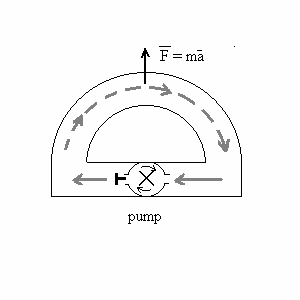Fig. 5

By analogy, for Biffeld-Brown effect in the capacitor, Figure 6, orbiting electrons of the dielectric are moving along curved trajectory if electric potential field exist between plates.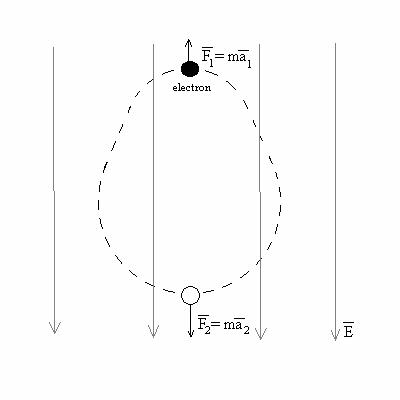Fig.6

So, nature of force "X" that was discovered in Biffeld-Brown effect is disclosed in this paper
as curved line motion acceleration that is a result of the asymmetrical centrifugal force that is created  in atoms of dielectric. Electric field of the capacitor transform normal trajectory of electrons in  asymmetrical one.  From this point of view, the Brown's force "X" is inertial phenomenon of non-linear mechanics and all pre-conditions are discussed in introduction of this paper are true for  Brown's electrogravitation systems.

Other Brown's patent number 3167206, USA, 1 June 1965 is example of asymmetry principle that allow to use the Coulomb's electrostatics forces to create the reactionless propulsion force. It is one of many ways to use the gradient of the field structure, that can be created both by means of gradient of the dielectrical properties and by means of the topology. Figure 7 is modified scheme of asymmetrical capacitor that can be created for non-reactive drive of space-craft.  Lines of intensity for electric potential field are perpendicular to surface and in strength of this reason the action-reaction forces of Coulomb electrostatic interaction are different.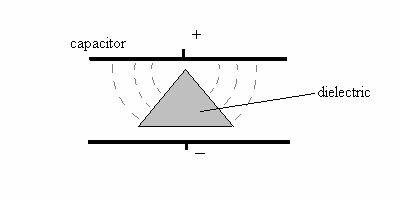Fig.7

The form of dielectric surface is the reason for forces asymmetry: vector sum of all forces is not
equal to zero in this case.

Asymmetry of Entropy

Other  method for reactionless motion is thermogravitation - application of thermal processes
inside of some closed system to create the motion of this system. Popular Science magazine vol. 126, 1935 describe some experiment, Figure 8. Two bodies can be moving in result of repulsion from each other. One body produce impact and other one produce impulse when bodies are reaching the ends of the rod. In result of this difference all device is moving in one side ( where interaction is "impulse").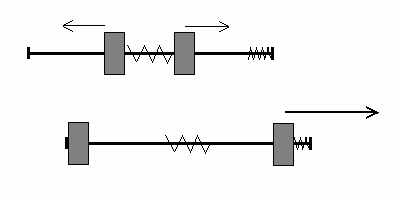Fig.8

Sure, this experiment is only non-periodical demonstration of the possibility to create
asymmetry of the energy transformation. To produce the second act of the repulsion between two masses, it is necessary to return masses to the central point of the device. It require some power (force action) and the question of symmetry for net work in left and in right parts of the device is open for discussion.

But experiment is fine example that allow to develop the idea: Non-reactive motion of closed system can be created as result of asymmetrical energy transformation.  The author's  version of the experiment was explained by next way: In this two-bodies  collision there is asymmetry of interaction. In one side there is elastic interaction (I used simple rubber  piece here) and kinetic energy of moving body is transmitted to the body of device. In second case  interaction is not elastic type interaction  but deformation mainly. Best version for it is absolute solid  body in this part of the device. So, in one side kinetic energy is transmitted but in other case kinetic  energy is transformed ( in heat ). Then, heat can be considered as chaotic motion result. So, in the  second case kinetic energy is transformed in kinetic chaotic energy. So, the difference is form of motion  only: ordered or non-ordered type of motion. If entropy notion is used for this case there is conclusion: gradient of entropy that is asymmetry of energy transformations is the reason for reactionless motion.

This conclusion allow to make the proposal for some scheme, Fig.9. I am grateful to  Academician V. I. Zubov, St.-Petersburg,  for useful discussion on this topic.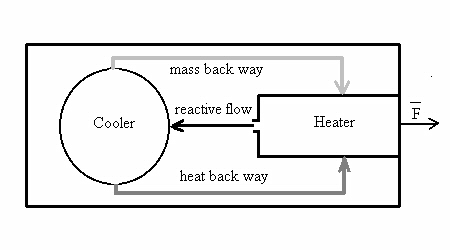Fig.9

The device that  use heater that create the reactive flow and cooler to transform the heat energy of this flow in other form of energy to return it in the heater. System can use reactive flow of mass in closed asymmetrical cycle to create the propulsion force. This flow is moving back from heater with some kinetic energy and at the moment the body of the device is getting some corresponding reactive impulse. But if velocity of steam flow is decreasing by means of heat absorption, the working mass (water, for example) is returned in collector of mass almost without opposite impulse since velocity of back-flow is small and kinetic energy is small also. The difference in right and left parts of the system is the different approach to the energy transformation: the right side of the system is using macro-interaction that is directed and ordered, total impulse is not zero vector, and the left part of the system is using micro-interactions at the molecular level and the total impulse vector can be made as zero or almost zero. The difference in total right and total left impulses produce the propulsion of the system and the temperature gradient inside of the system. It is possible to say the system is moving by means of the inner temperature gradient.

Note, that many ancient Indian papers described  flying device that use mercury in closed cycle
to produce the propulsion force.

Conclusion

Taking into consideration that the entropy function is close connected with direction of time flow, the asymmetry of this function in different parts of moving system is base for supposition: Any "reactionless" drive produce propulsion force in space that is paired with time directed force.
The  Newton's  Law can be generalized: Forces can be created in pair only, and any active force have spatial or chronal  equal and opposite  reactive force.

By this version of known law there is some pre-conditions  for new technologies:  local control by rate of time. The Law of conservation for quantity of the motion is true for inertia-less reaction-less systems. We can consider  new type of the motion: motion in time that is subordinated to this law also. For this mathematics it is necessary to use time directed four-dimensional vectors of impulse, force, electric field intensity and so on.

References
1. Newton I, Letter to Boile of 1679, "Isaak  Newton", Acad. Vavilov S.I., published by The Academy of the Sciences of the USSR, Moscow-Leningrad, 1943, in russian, p.95-98.
2. Sakharov A., Vacuum Quantum Fluctuations in Curved Space and the Theory of Gravitation, Soviet Physics  Doclady, Vol. 12, No.11, 1040-1041, 1968.
3. H.Puthoff, "Gravity as a Zero-Point-Fluctuation Force", Phys. Rew. A, 39, 2333-2342, 1989b.
4.  B.Haisch, "Zero-Point-Field-Induced Inertia and Gravitation: Questions, Answers and Issues", Department 91-30, Building 252, Lockheed Palo Alto Research Laboratory, 3251 Hanover St., Palo Alto CA 94304 USA.
5.  B.Haisch, A.Rueda and H.E.Puthoff, "Beyond E=mc2", The Science, November/December 1994, Vol.34/ No.6, p.26.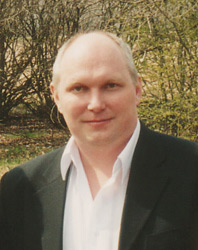a2509@yahoo.com